# Petra on Programming: The Correlation Cycle Indicator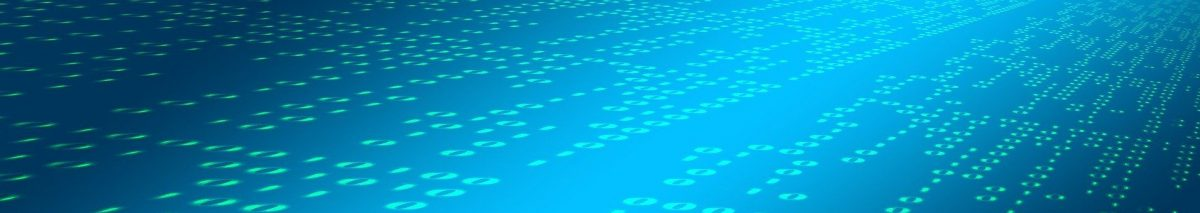The previous article dealt with indicators based on correlation with a trend line. This time we’ll look into another correlation-based indicator by John Ehlers. The new Correlation Cycle indicator (CCY) measures the price curve correlation with a sine wave. This works surprisingly well – not for generating trade signals, but for a different purpose.

Ehlers published the indicator together with TradeStation code in the recent S&C magazine. Since the C language supports function pointers, we can code it in a shorter and more elegant way:

```var correlY(var Phase); // function pointer
var cosFunc(var Phase) { return cos(2*PI*Phase); }
var sinFunc(var Phase) { return -sin(2*PI*Phase); }

var correl(vars Data, int Length, function Func)
{
correlY = Func;
var Sx = 0, Sy = 0, Sxx = 0, Sxy = 0, Syy = 0;
int count;
for(count = 0; count < Length; count++) {
var X = Data[count];
var Y = correlY((var)count/Length);
Sx += X; Sy += Y;
Sxx += X*X; Sxy += X*Y; Syy += Y*Y;
}
if(Length*Sxx-Sx*Sx > 0 && Length*Syy-Sy*Sy > 0)
return (Length*Sxy-Sx*Sy)/sqrt((Length*Sxx-Sx*Sx)*(Length*Syy-Sy*Sy));
else return 0;
}

var CCY(vars Data, int Length) { return correl(Data,Length,cosFunc); }var CCYROC(vars Data, int Length) { return correl(Data,Length,sinFunc); }```

The correl function measures the correlation of the Data series with an arbitrary curve given by the Func function. This allows us to create all sorts of correlation indicators by just using a different Func. For example, it reduces Ehlers’ Correlation Trend Indicator from the previous article to 2 lines:

```var trendFunc(var Phase) { return -Phase; }

var CTI(vars Data,int Length) { return correl(Data,Length,trendFunc); }
```

The empty correlY function pointer in the code above serves as a template for Func, and is used for calling it inside the correl function. For the CTI it’s simply a rising slope (negative because series are in reverse order), for the CCY it’s the standard cosine function.

At first let’s see how the CCY indicator behaves when applied to a sine wave. We’re using Zorro’s wave generator to produce a sine chirp with a rising cycle length from 15 up to 30 bars, which is 25% below and 50% above the used CCY period of 20 bars. The code:

```function run(){   MaxBars = 300;   LookBack = 40;   asset(""); // dummy asset   ColorUp = ColorDn = 0; // don't plot a price curve      vars Chirp = series(genSine(15,30));   plot("Chirp",2*Chirp-1,LINE,BLUE);   plot("CCY",CCY(Chirp,20),LINE,RED);}
```

And the result: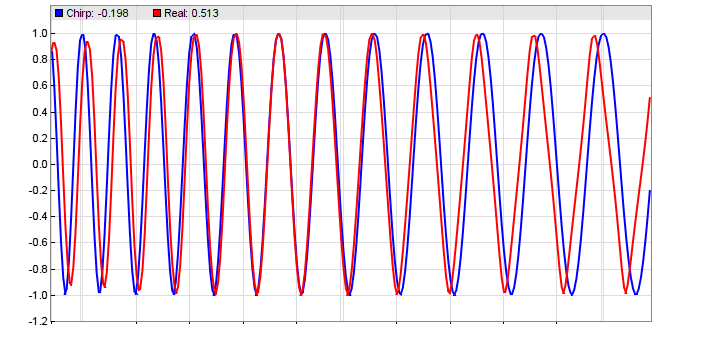This confirms Ehlers’ stress test. A shorter period results in a phase lag, a longer period in a phase lead. We’re now going to apply the indicator to real-world price curves. This code displays the CCY and its rate of change (CCYROC) in a SPY chart:

```function run(){   BarPeriod = 1440;
LookBack = 40;
StartDate = 20190101;

asset("SPY");

vars Prices = series(priceClose());
plot("CCY(14)",CCY(Prices,14),NEW|LINE,RED);
plot("ROC(14)",CCYROC(Prices,14),LINE,GREEN);
}```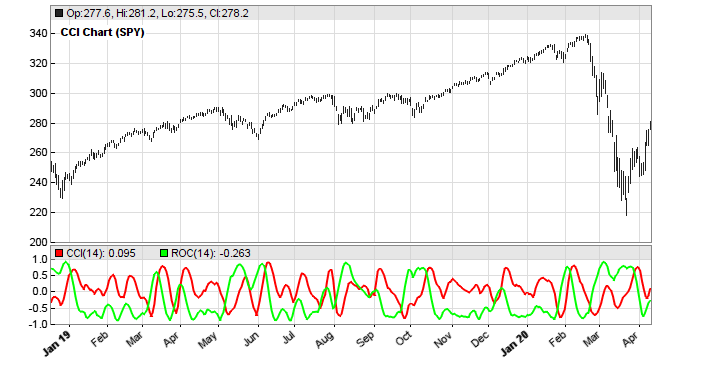What’s the use of the Correlation Cycle indicator in a trading system? The chart might hint that its peaks or valleys could be used for trade signals, but you can save the time of testing it: I did already. The CCY is no good for trade signals. But Ehlers had another idea. The phase angle of the CCY and CCYROC reflects the market state. It returns 1 for a rising trend, -1 for a falling trend, and 0 for cycle regime. Here’s the code of Ehlers CCY market state indicator:

`var CCYState(vars Data,int Length,var Threshold){   vars Angles = series(0,2);   var Real = correl(Data,Length,cosFunc);   var Imag = correl(Data,Length,sinFunc);// compute the angle as an arctangent function and resolve ambiguity   if(Imag != 0) Angles = 90 + 180/PI*atan(Real/Imag);   if(Imag > 0) Angles -= 180;// do not allow the rate change of angle to go negative   if(Angles-Angles < 270 && Angles < Angles)      Angles = Angles;   //return Angles;// compute market state   if(abs(Angles-Angles) < Threshold)      return ifelse(Angles < 0,-1,1);   else return 0;}`

Applied to SPY: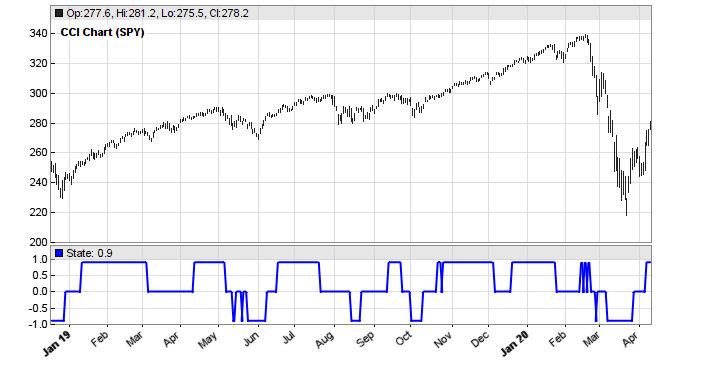At first glance, trends and cycles seem to be rather well and timely detected. But how useful is the indicator in a real trading system?

For finding out, we’ll compare the performance with and without market state detection of a simple trend follower, as in the first Zorro workshop. It uses a lowpass filter for detecting trend reversals. The only parameter is the cutoff period of the low pass filter. This parameter is walk forward optimized, so the system does depend on any choosen parameter value. The trend follower without market state detection:

```function run()
{
set(PARAMETERS);
BarPeriod = 1440;
LookBack = 40;
NumYears = 8;

asset("SPY");

NumWFOCycles = 4;
int Cutoff = optimize(10,5,30,5);

vars Prices = series(priceClose());
vars Signals = series(LowPass(Prices,Cutoff));
if(valley(Signals))
enterLong();
else if(peak(Signals))
enterShort();
}```

The system enters a long position on any valley of the lowpass filtered price curve, and a short position on any peak. The resulting equity curve: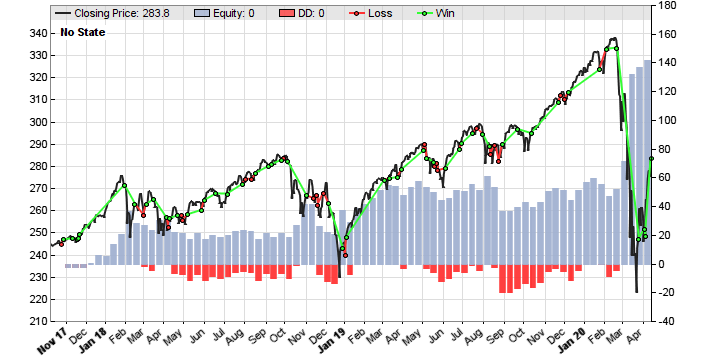We can see that the simple SPY trend follower is not very good. Yes, it’s profitable, but the main profit came from some lucky trades at the corona drop. In the years before the system had long flat periods. Let’s see if the CCYState indicator can help. Its two parameters, period and threshold, are also walk forward optimized. The new script:

```function run()
{
set(PARAMETERS);
BarPeriod = 1440;
LookBack = 40;
NumYears = 8;

asset("SPY");

NumWFOCycles = 4;
int Cutoff = optimize(10,5,30,5);
int Period = optimize(14,10,25,1);
var Threshold = optimize(9,5,15,1);

vars Prices = series(priceClose());
var State = CCYState(Prices,Period,Threshold);
plot("State",State*0.9,NEW|LINE,BLUE);
vars Signals = series(LowPass(Prices,Cutoff));

if(State != 0) {
if(valley(Signals))
enterLong();
else if(peak(Signals))
enterShort();
}
else {
exitLong();
exitShort();
}
}```

The new system trades only when the market state is 1 or -1, indicating trend regime. It goes out of the market when the market state is 0. We can see that this improves the equity curve remarkably: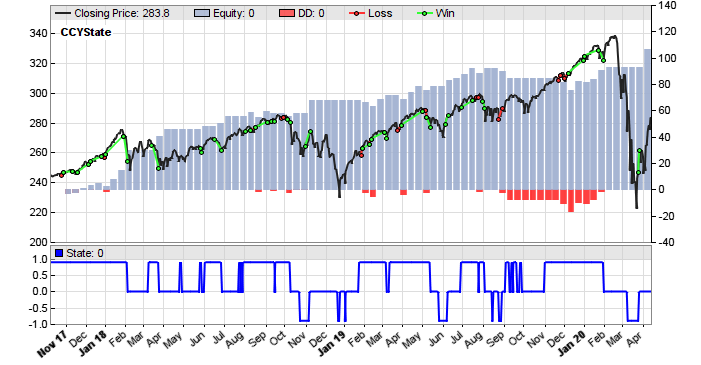I think most people would prefer this system to the previous one, even though it stayed out of the market at the corona drop. Ehlers’ market state indicator did a good job.

### Reference

John Ehlers, Correlation Cycle Indicator, Stocks&Commodities 6/2020

The indicators and trade systems are available in the Scripts 2020 repository.

## 12 thoughts on “Petra on Programming: The Correlation Cycle Indicator”

1.trader says:

it fits well into certain market conditions in sinusoidal phase.But other times it fells apart.

2.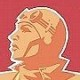Petra Volkova says:

Thats why you better not use it for trade signals, but for market state detection.

3.James says:

Hey Petra,

What is the ROC function used? It looks bounded vs the formula Tradingview uses:
ROC = [(CurrentClose – Close n periods ago) / (Close n periods ago)] X 100

thanks

4.Petra Volkova says:

The ROC of something is its first derivative. d/dx cos(x) = -sin(x).

5.Hans says:

Hi Petra,

I wonder how stable a system based on this could be when adding an additional signal-/entry- and position management system for the cyclic phases as well….

cheers

6.Richard says:

Any chance we could see the full code in one entry? Zorro does not have this CCYState indicator. many thanks.

7.jcl says:

The full code should be in the Scripts 2020 archive – Petra adds all new code in there.

8.hast29 says:

Hi Petra, I have reproduced your experiment and there is one strange thing in the code. The script with the State filter actually does not care, in which direction the trade is beeing opened. I mean there are short trades for State >0 and long trades for State <0. Does not this contradict the Ehlers paper ? Many thanks …

9.Petra Volkova says:

Right, it only filters by market state. The direction is by signal peaks and valleys. I remember that I tried additional filtering by market direction, but it did not improve the system further. Anyway you’re free to test it.

10.Jaroslav Vojtechovsky says:

Yes, I changed the State detector to the „directional“ version and indeed, no positive effect. Which is bothering me a bit, because the explanation of Ehlers was strictly „directional“.
I will certainly make more tests based on your valuable work and the new indicator code. For example there is a possibility to use the Correlation Trend Indicator from your last blog also as the State detector …
Many thank for the code and your work !!

11.Spyros says:

Just a few thoughts from a newbie:
You can improve the system profitability by excluding exitLong/Short when State goes back to zero and by adding the condition to Buy/Sell only when the price is over/under an optimized SMA. You also have to optimize a TakeProfit target. I just tried it to GBP/USD and it is a beauty!

EHLERS compiling…………